# 3. Let I be a left ideal of R and let (: R) reRRCI (a) : R) is an ideal of R. If l is regular, th...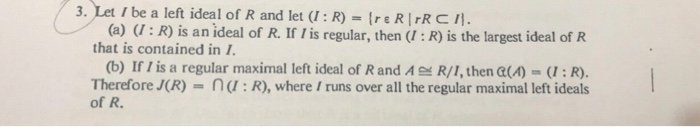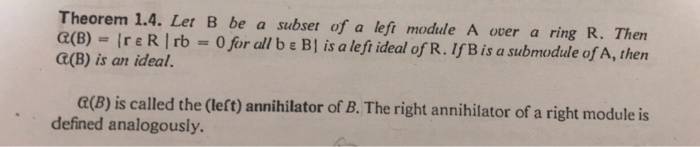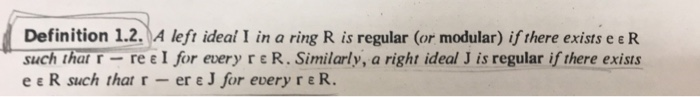3. Let I be a left ideal of R and let (: R) reRRCI (a) : R) is an ideal of R. If l is regular, then (: R) is the largest ideal of R that is contained in 1 (b) If I is a regular maximal left ideal of Rand AR/I, then (A(R). Therefore J(R) na:R), where /runs over all the regular maximal left ideals of R.
Theorem 1.4. Let B be a subset of a left module A over a ring R. Thern a(B)-Ire R | rb-0 for all b ε BI is a left ideal of R. If B is a submodule of A, then C(B) is an ideal. e(B) is called the (left) annihilator of B. The right annihilator of a right module is defined analogously.
Definition 1.2.A left ideal I in a ring R is regular (or modular) if there exists e e R suchthat-r-re ε| for every r ε R. Similarly, a right ideal J is regular if there exists e e . R such that r-ere J for every re R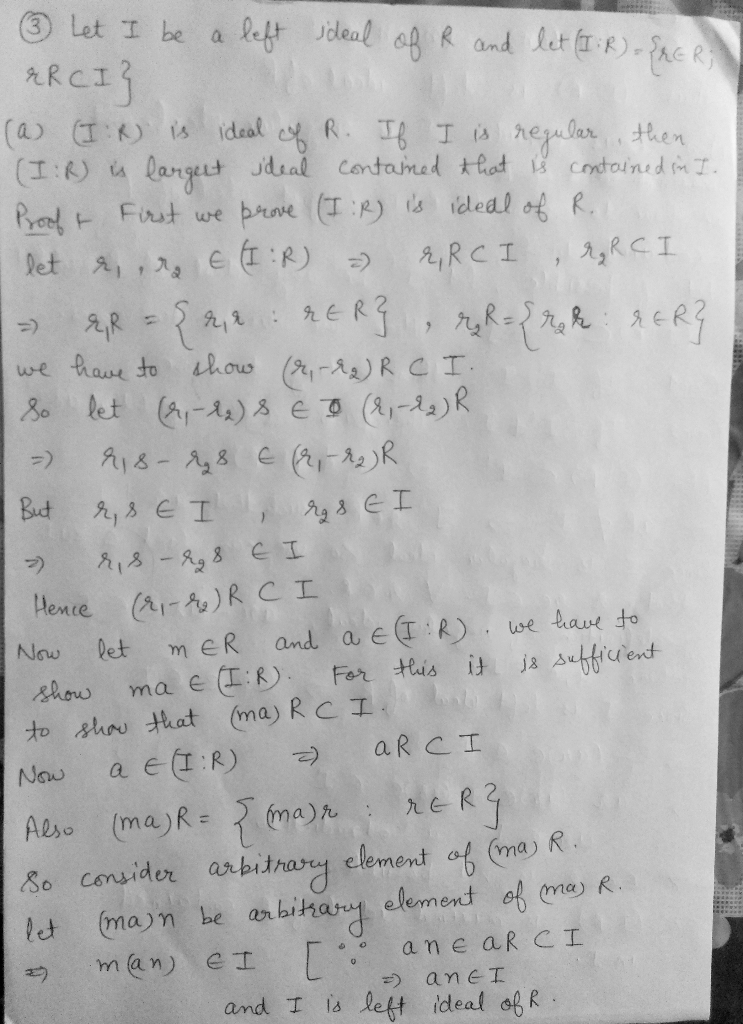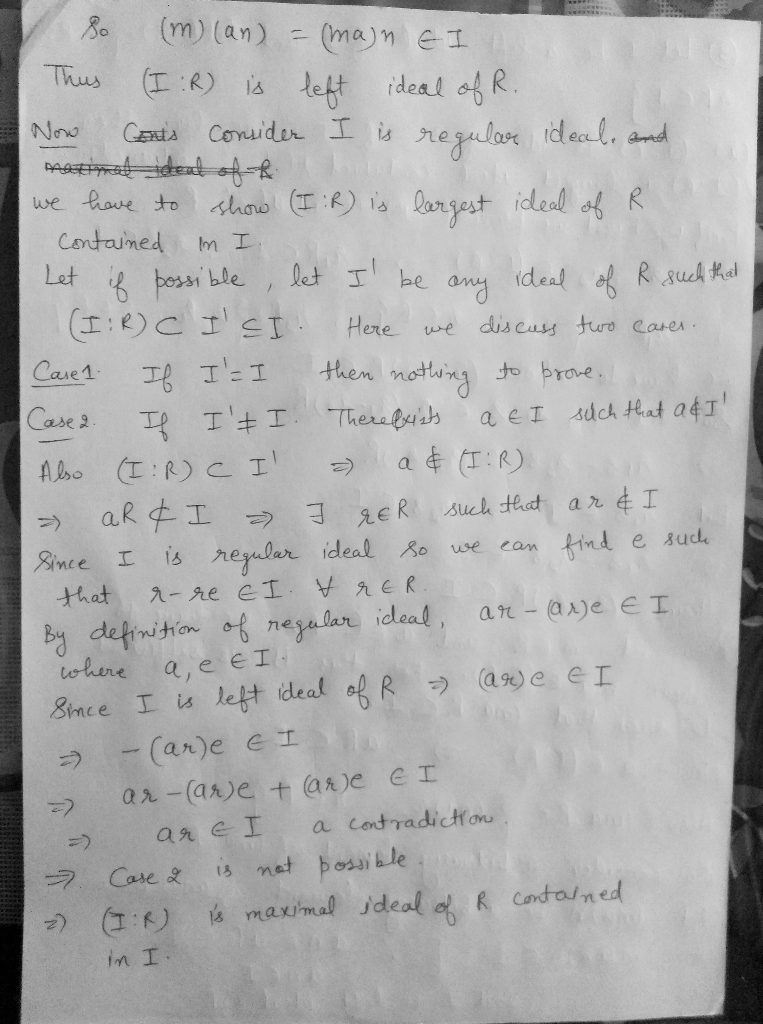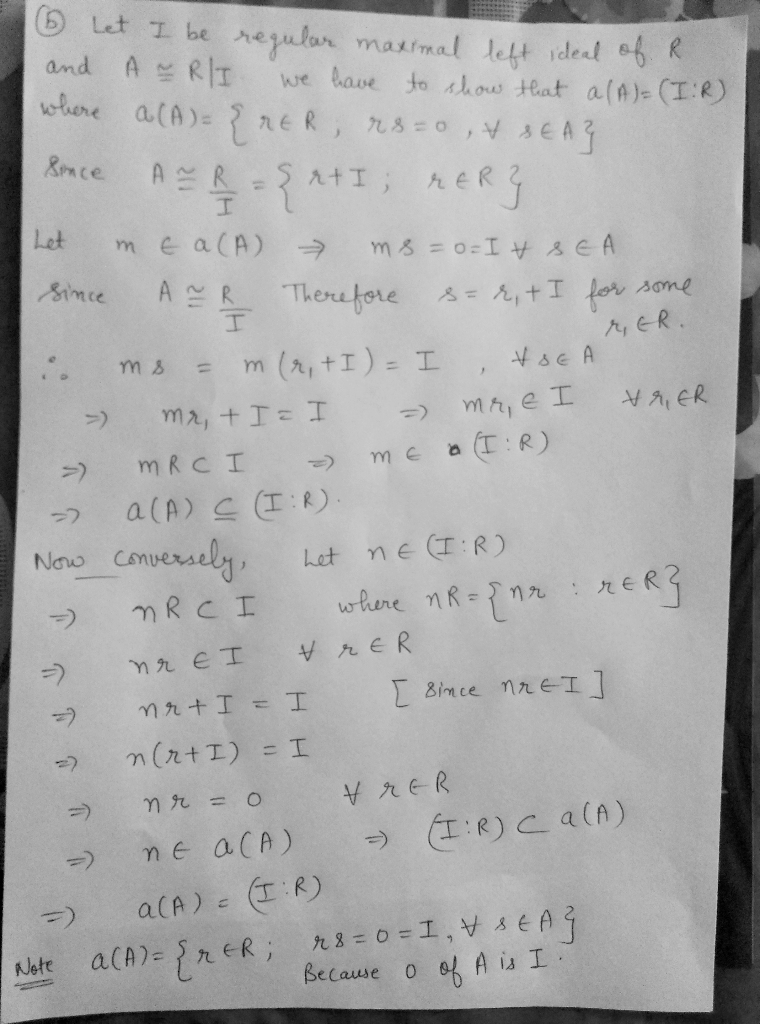##### Add Answer of: 3. Let I be a left ideal of R and let (: R) reRRCI (a) : R) is an ideal of R. If l is regular, th...
More Homework Help Questions Additional questions in this topic.

• #### For the following languages, state if they are regular, context free but not regular, or not...

Need Online Homework Help?